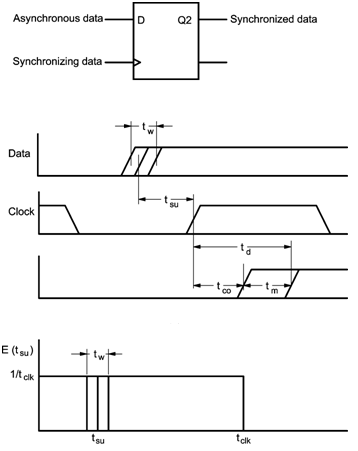Home > finite state machines > Metastability

# Metastability

Metastability is a type of failure which occurs when digital circuits attempt to synchronize asynchronous digital data. As shown in Figure, when a clocked flip-flop synchronizes an asynchronous input there is a small probability that the output exhibits an unpredictable response. This phenomenon occures when the input transition changes the the setup and hold time specification. This occurs within the small timing window where the flip-flop accepts the inputs. Thus, under this kind of circumstances the flip-flop enters in an unstable intermediate state which is called as called metastable state. In this case a slight deviation leads to the outputs to revert to one of the two stable states. However, the settling time depends not only on the gain bandwidth product of the circuit, but also on the original balance and the noise level of the circuit. As shown in Figure, a critical trigger window tW is defined. If a flip-flop timing constraint is changed during the time window tW, the output Q is still unresolved within a decision time td. Referenced to the beginning of the trigger event which is the positive clock edge for the sample circuit in Figure, td thus includes the normal propagation delay tCO and extra delay due to metastability tM. The relation tW = f(td) is used for defining the metastable behavior of flipflops.The exponential function is asymptotically valid for the relation as follows, tW = f(td) = a*exp(b*td) â€¢ The probability density function for actual setup time E(tsu) is unity for one clock period tCLK . The probability for one data event to hit the critical window tW is equivalent to the indicated area tW/tCLK. With given tW(td) the reliability mean time between failures, (MTBF) of a synchronizer is calculated as follows, MTBF(td) = 1/(tW(td)*fCLK*fDATA)

This expression can also be rewritten in exponential form as, MTBF = exp(td/k2)/(k1 *fCLK*fDATA) Where k1 and k2 are constants which will get from exponential results to calculate MTBF with relative td, clock frequency fCLK and data frequency fDATA.